# Introduction of Structured Learning Hungyi Lee Structured Learning

• Slides: 66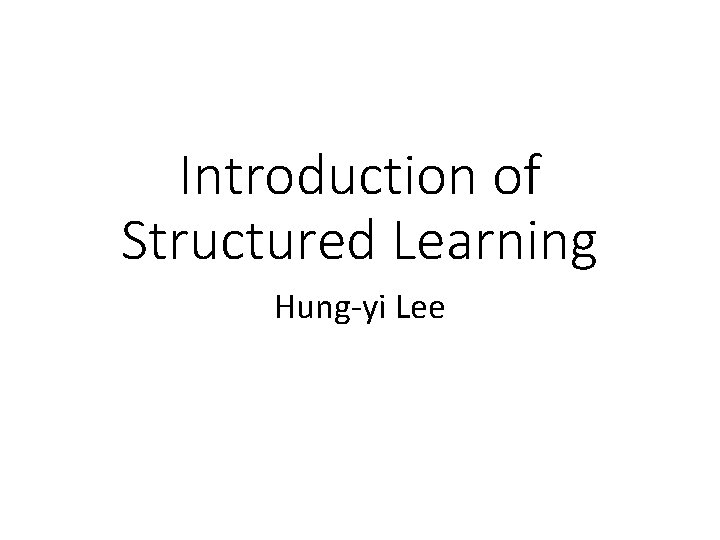Introduction of Structured Learning Hung-yi Lee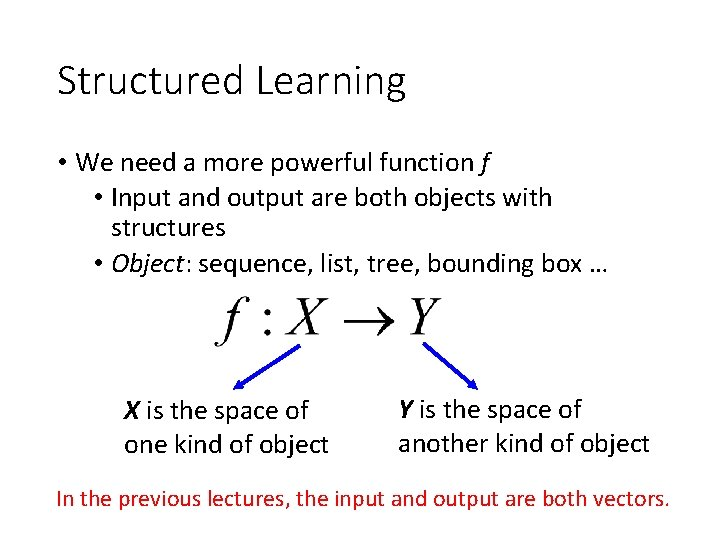Structured Learning • We need a more powerful function f • Input and output are both objects with structures • Object: sequence, list, tree, bounding box … X is the space of one kind of object Y is the space of another kind of object In the previous lectures, the input and output are both vectors.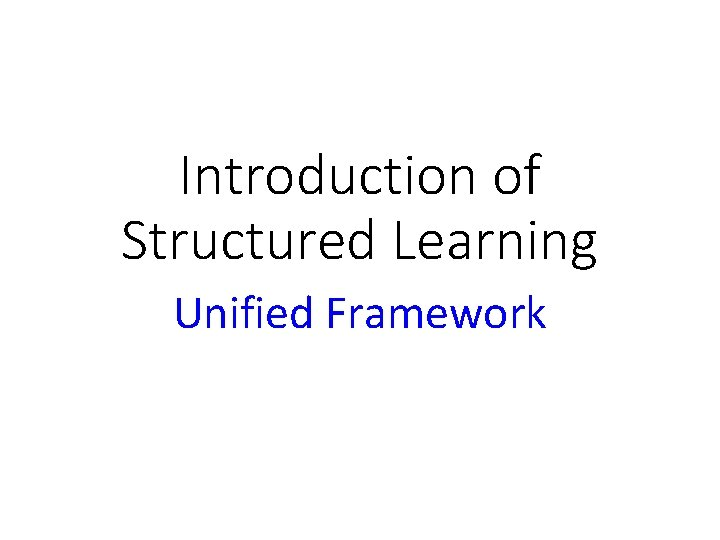Introduction of Structured Learning Unified Framework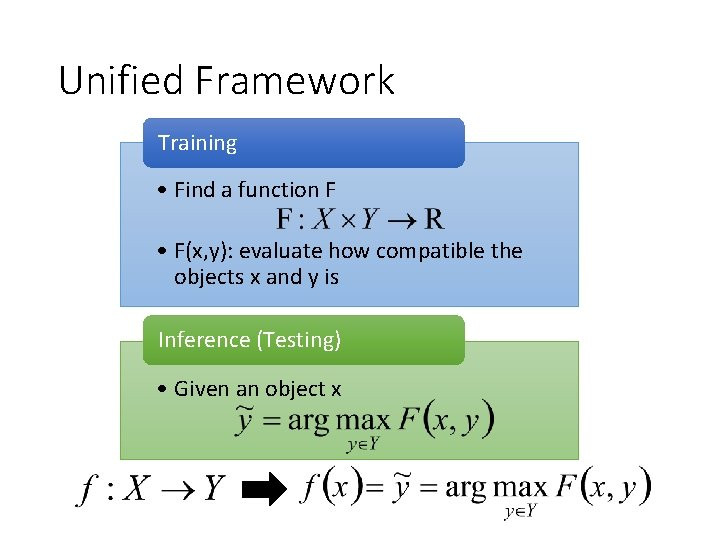Unified Framework Training • Find a function F • F(x, y): evaluate how compatible the objects x and y is Inference (Testing) • Given an object x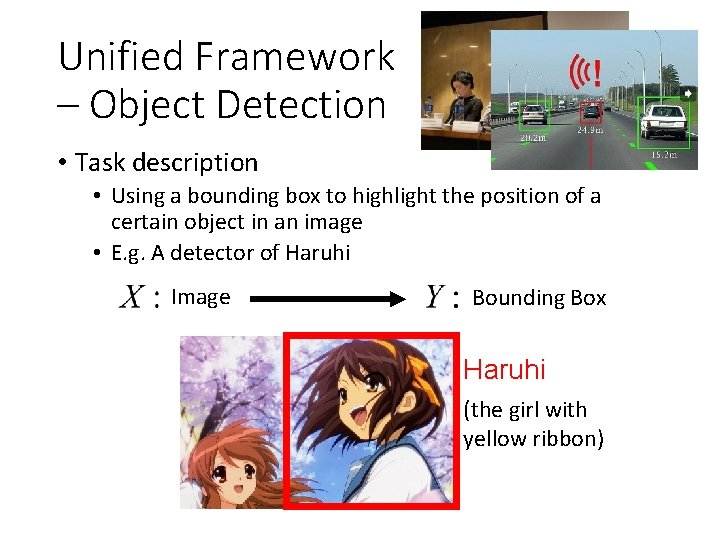Unified Framework – Object Detection • Task description • Using a bounding box to highlight the position of a certain object in an image • E. g. A detector of Haruhi Image Bounding Box Haruhi (the girl with yellow ribbon)Unified Framework – Object Detection x: Image y: Bounding Box F(x, y) F( ) the correctness of taking range of y in x as “Haruhi” F(x, y)Unified Framework – Object Detection (output result) F(x, y) 10 3 2 …… input x = Enumerate all possible bounding box y -1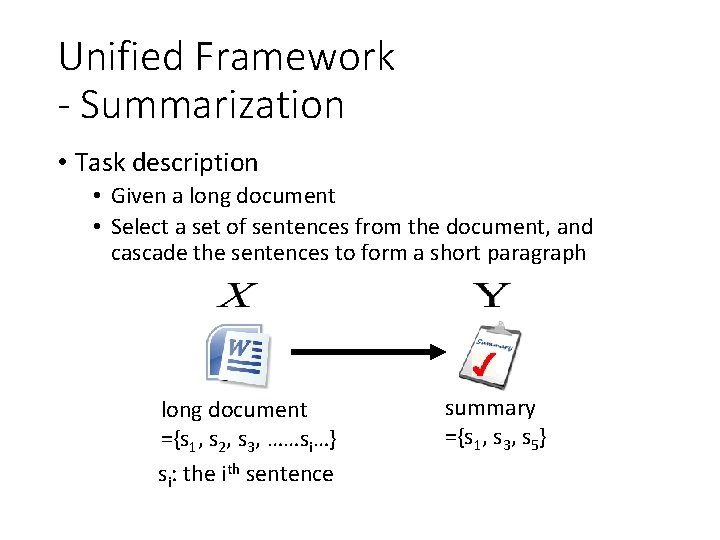Unified Framework - Summarization • Task description • Given a long document • Select a set of sentences from the document, and cascade the sentences to form a short paragraph long document ={s 1, s 2, s 3, ……si…} si: the ith sentence summary ={s 1, s 3, s 5}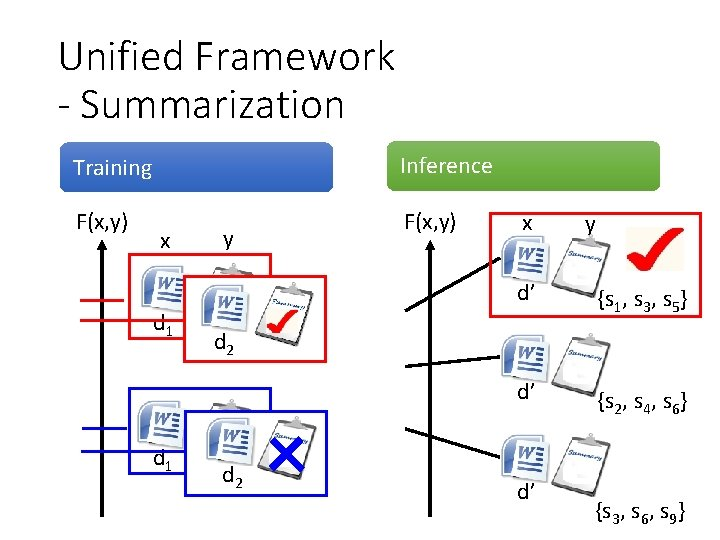Unified Framework - Summarization Training Inference F(x, y) x d 1 y x y d’ {s 1, s 3, s 5} d’ {s 2, s 4, s 6} d 2 d’ {s 3, s 6, s 9}Unified Framework - Retrieval • Task description • User input a keyword Q • System returns a list of web pages “Obama” (keyword ) d 10011 d 98776 …… A list of web pages (Search Result)Unified Framework - Retrieval Training F(x, y) Inference F(x, y) d 103 x=“Obama”, y= d 300 d 103 x=“Trump”, y=…… d 300 …… d 666 x=“Obama”, y= d 444 d 133 …… d 220 x=“Trump”, y= …… d 203 x=“Haruhi”, y= d 330 …… d 103 x=“Haruhi”, y= d 304 …… d 103 x=“Haruhi”, y= d 305 ……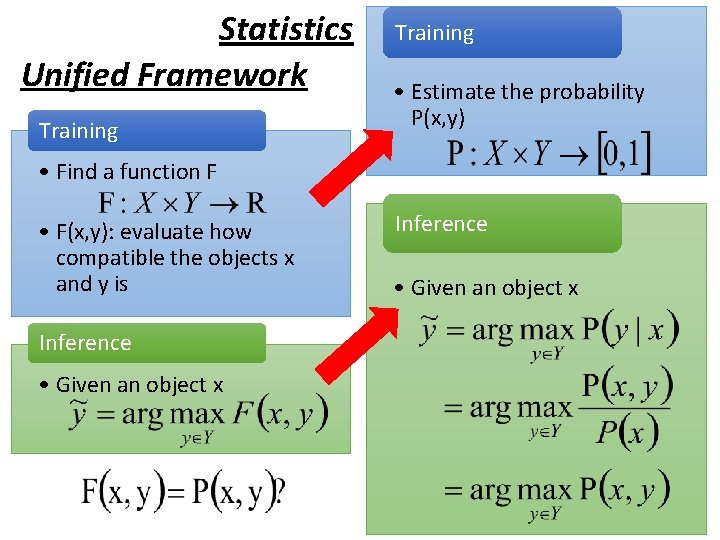Statistics Unified Framework Training • Estimate the probability P(x, y) • Find a function F • F(x, y): evaluate how compatible the objects x and y is Inference • Given an object x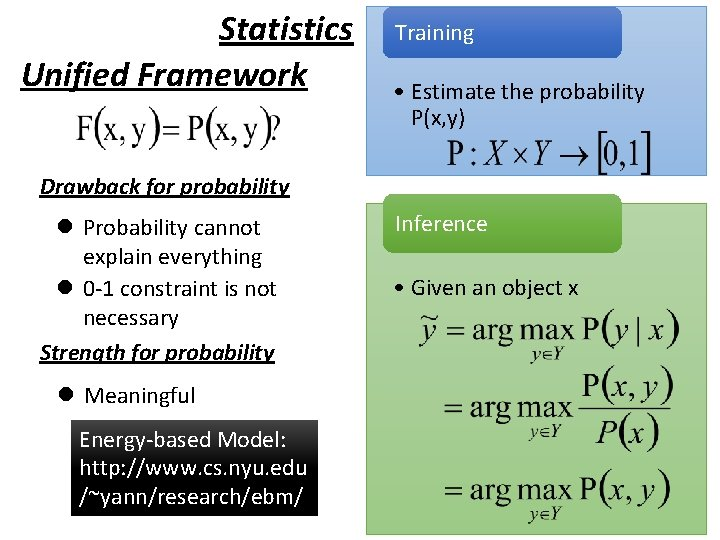Statistics Unified Framework Training • Estimate the probability P(x, y) Drawback for probability l Probability cannot explain everything l 0 -1 constraint is not necessary Strength for probability l Meaningful Energy-based Model: http: //www. cs. nyu. edu /~yann/research/ebm/ Inference • Given an object x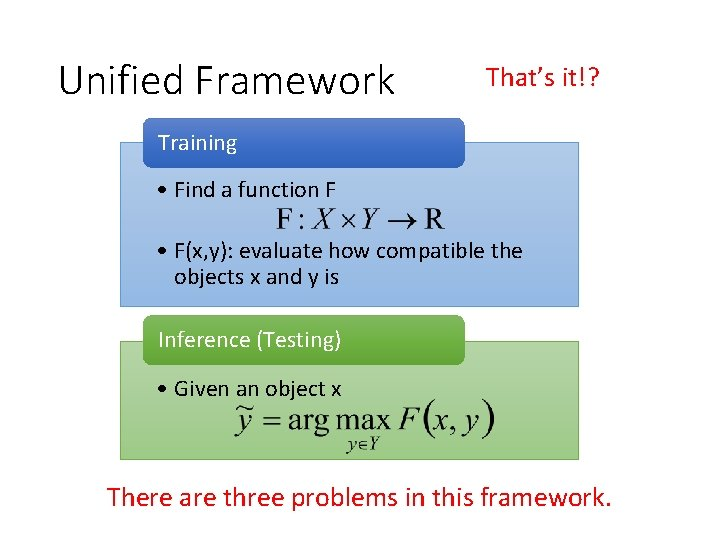Unified Framework That’s it!? Training • Find a function F • F(x, y): evaluate how compatible the objects x and y is Inference (Testing) • Given an object x There are three problems in this framework.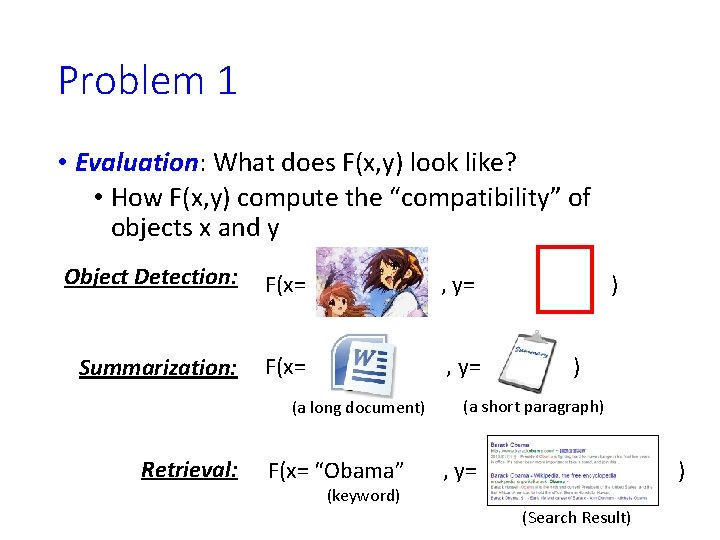Problem 1 • Evaluation: What does F(x, y) look like? • How F(x, y) compute the “compatibility” of objects x and y Object Detection: F(x= , y= Summarization: F(x= , y= (a long document) Retrieval: F(x= “Obama” (keyword) ) ) (a short paragraph) , y= ) (Search Result)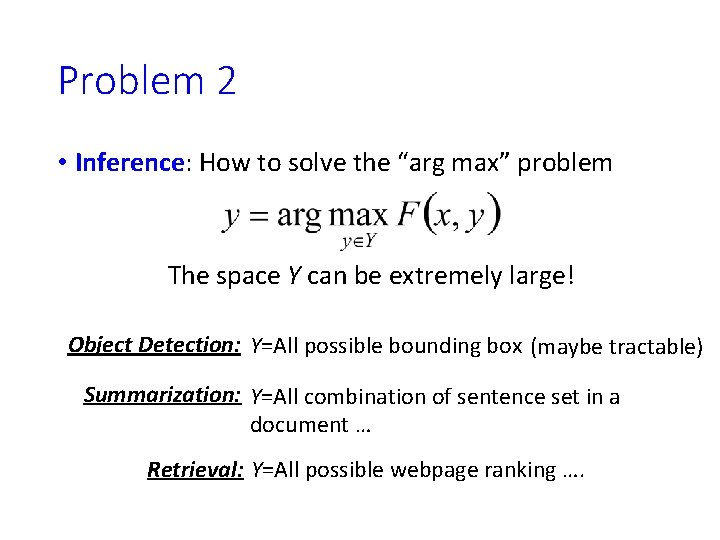Problem 2 • Inference: How to solve the “arg max” problem The space Y can be extremely large! Object Detection: Y=All possible bounding box (maybe tractable) Summarization: Y=All combination of sentence set in a document … Retrieval: Y=All possible webpage ranking ….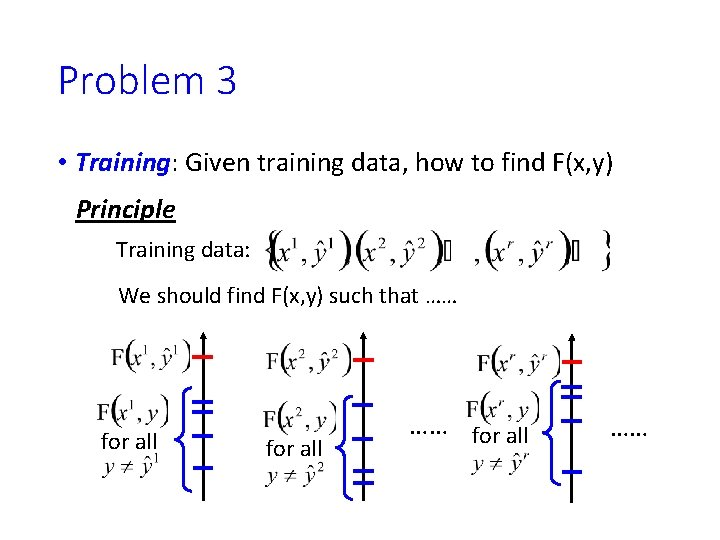Problem 3 • Training: Given training data, how to find F(x, y) Principle Training data: We should find F(x, y) such that …… for all ……Three Problems Problem 1: Evaluation • What does F(x, y) look like? Problem 2: Inference • How to solve the “arg max” problem Problem 3: Training • Given training data, how to find F(x, y)Have you heard the three problems elsewhere? From 數位語音處理Link to DNN? Training The same as what we have learned. Inference In handwriting digit classification, there are only 10 possible y. N(x) … DNN y = [ 1 0 0 0 …… ] y = [ 0 1 0 0 …… ] y = [ 0 0 1 0 …… ] Find max F(x, y)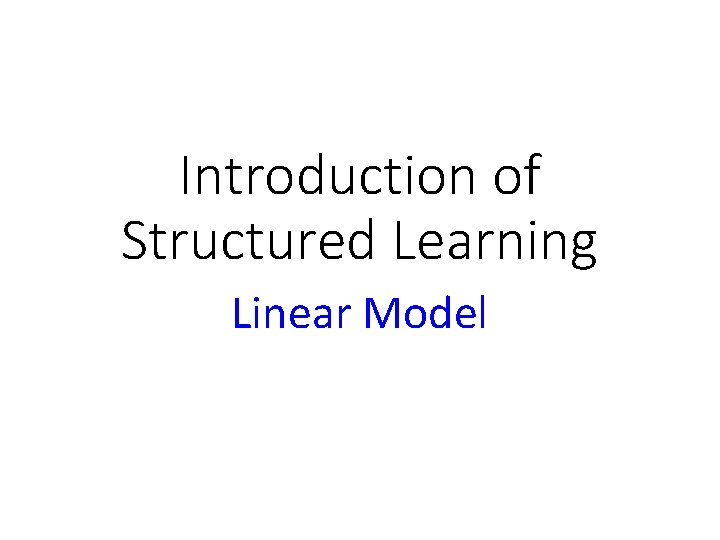Introduction of Structured Learning Linear ModelStructured Linear Model Problem 1: Evaluation • What does F(x, y) look like? in a specific form Problem 2: Inference • How to solve the “arg max” problem Problem 3: Training • Given training data, how to find F(x, y)Structured Linear Model: Problem 1 • Evaluation: What does F(x, y) look like? Characteristics x y Learning from data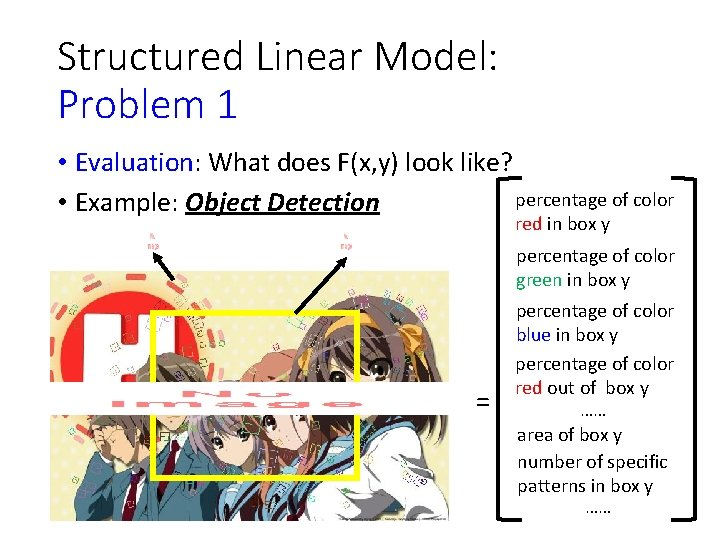Structured Linear Model: Problem 1 • Evaluation: What does F(x, y) look like? percentage of color • Example: Object Detection red in box y percentage of color green in box y = percentage of color blue in box y percentage of color red out of box y …… area of box y number of specific patterns in box y ……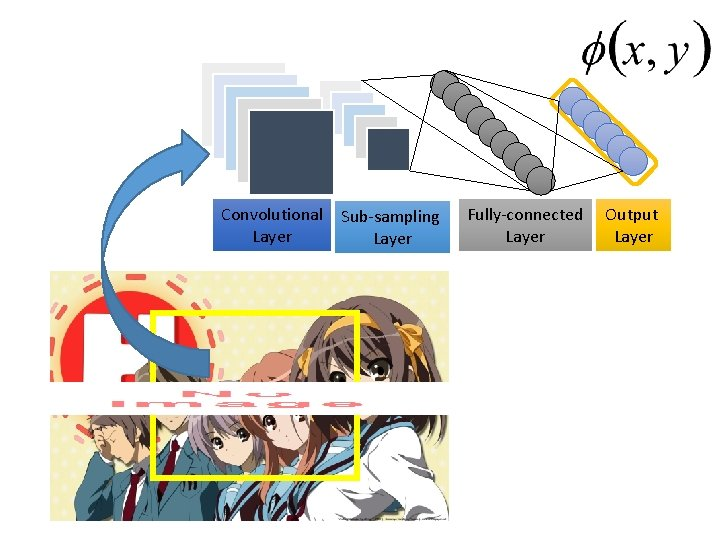Convolutional Layer Sub-sampling Layer Fully-connected Layer Output Layer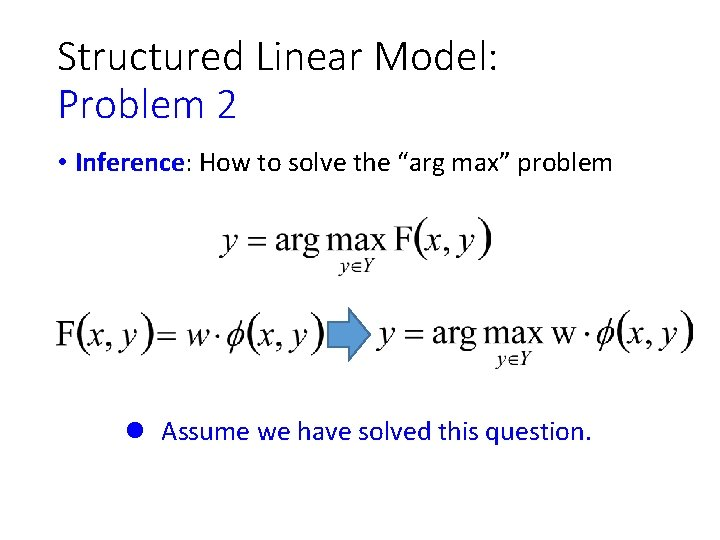Structured Linear Model: Problem 2 • Inference: How to solve the “arg max” problem l Assume we have solved this question.Structured Linear Model: Problem 3 • Training: Given training data, how to learn F(x, y) • F(x, y) = w·φ(x, y), so what we have to learn is w Training data: We should find w such that (All training examples) (All incorrect label for r-th example)Structured Linear Model: Problem 3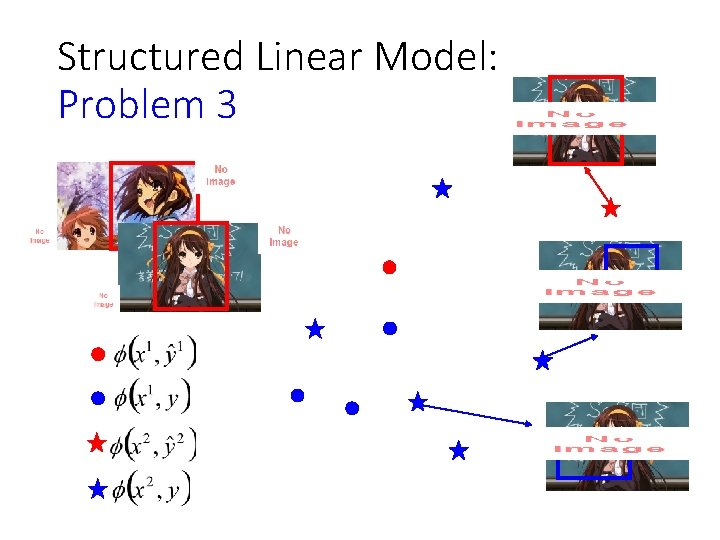Structured Linear Model: Problem 3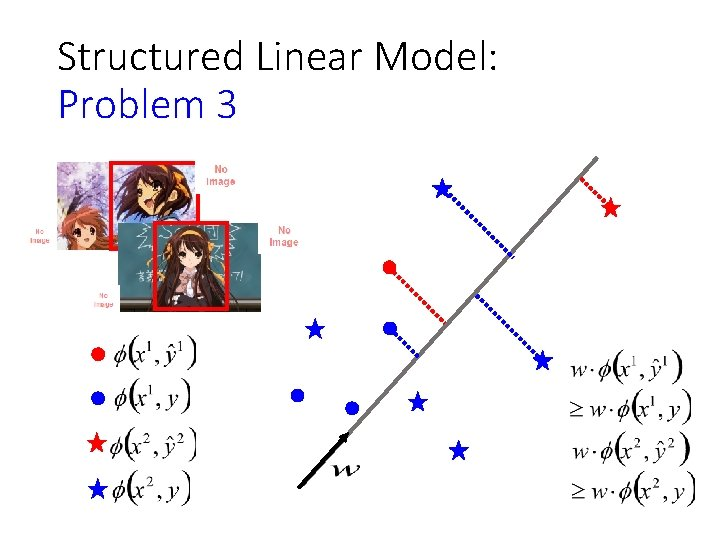Structured Linear Model: Problem 3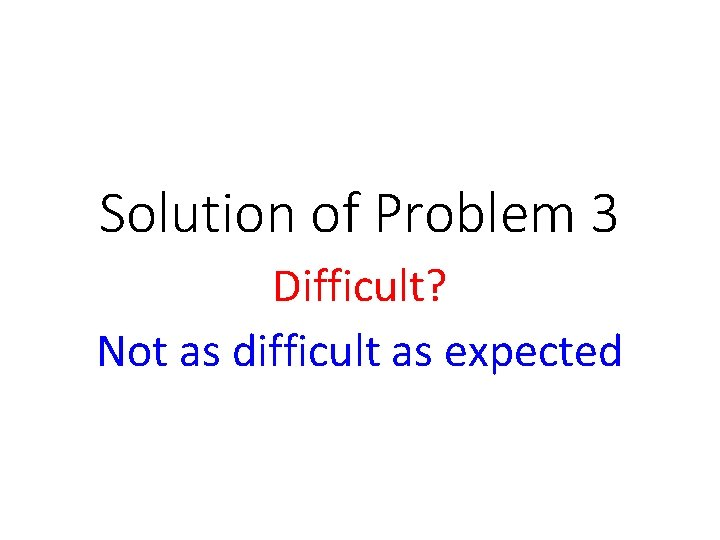Solution of Problem 3 Difficult? Not as difficult as expected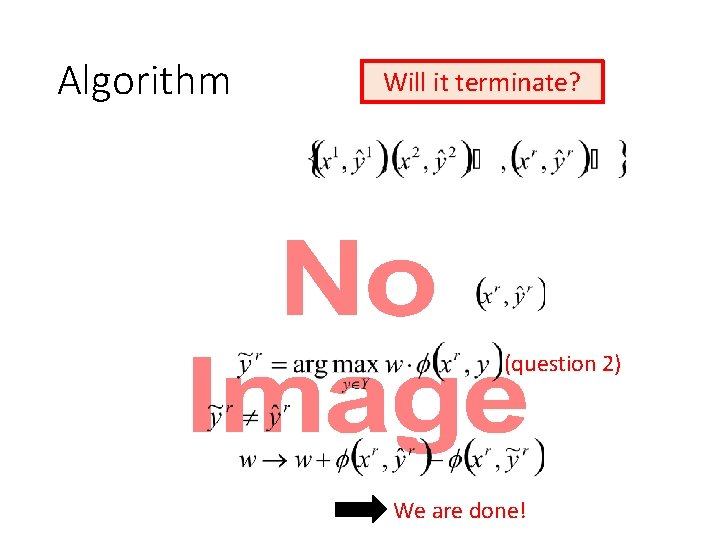Algorithm Will it terminate? • (question 2) We are done!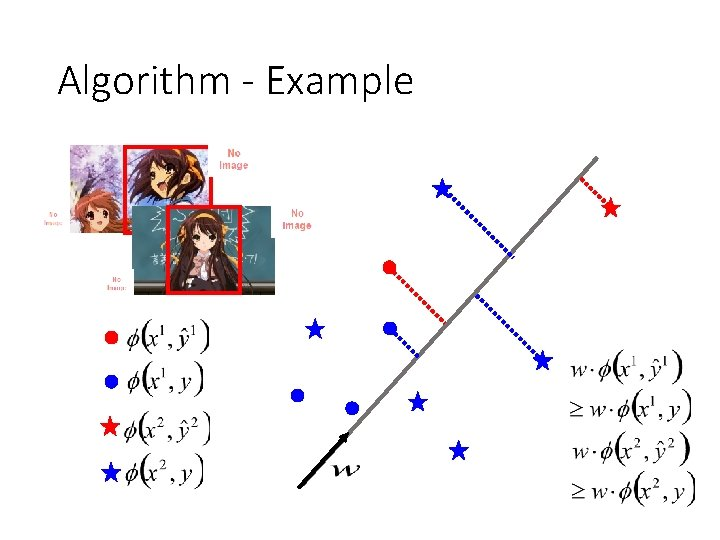Algorithm - Example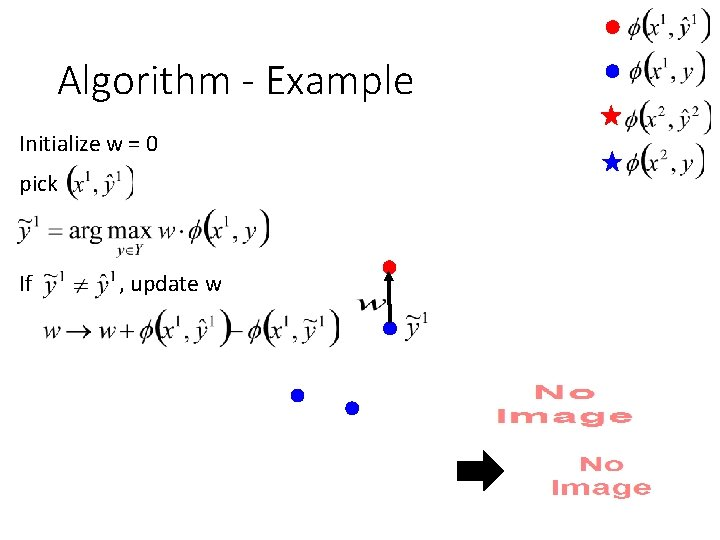Algorithm - Example Initialize w = 0 pick If , update w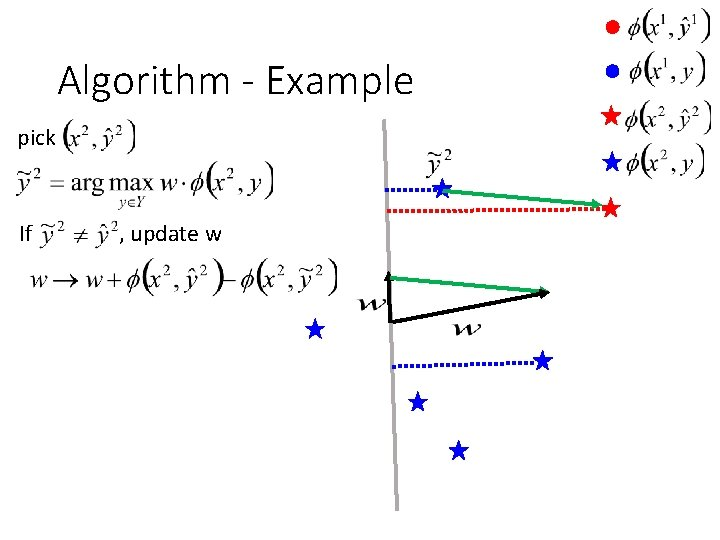Algorithm - Example pick If , update wAlgorithm - Example pick again do not update w So we are doneAssumption: Separable • (All training examples) (All incorrect label for an example) (The target exists)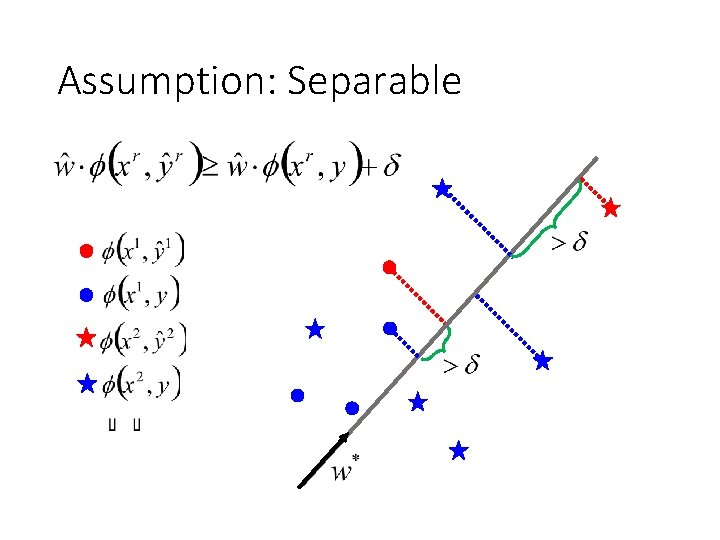Assumption: Separable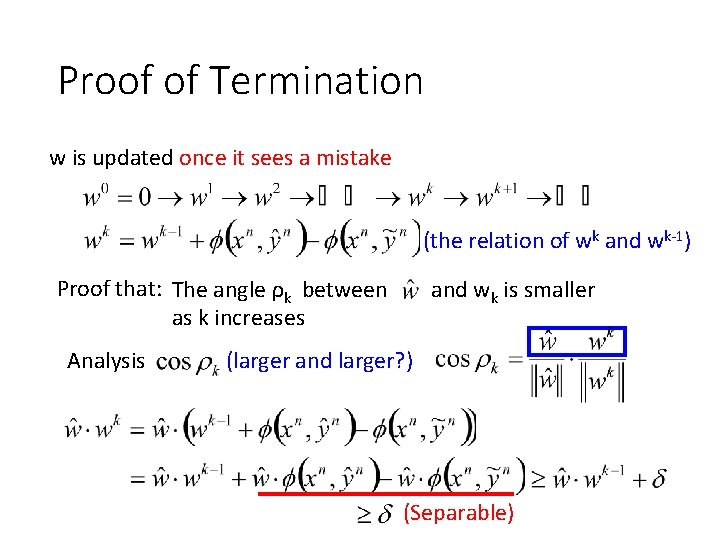Proof of Termination w is updated once it sees a mistake (the relation of wk and wk-1) Proof that: The angle ρk between as k increases Analysis and wk is smaller (larger and larger? ) (Separable)Proof of Termination w is updated once it sees a mistake (the relation of wk and wk-1) Proof that: The angle ρk between as k increases Analysis and wk is smaller (larger and larger? ) =0 ≥δ (so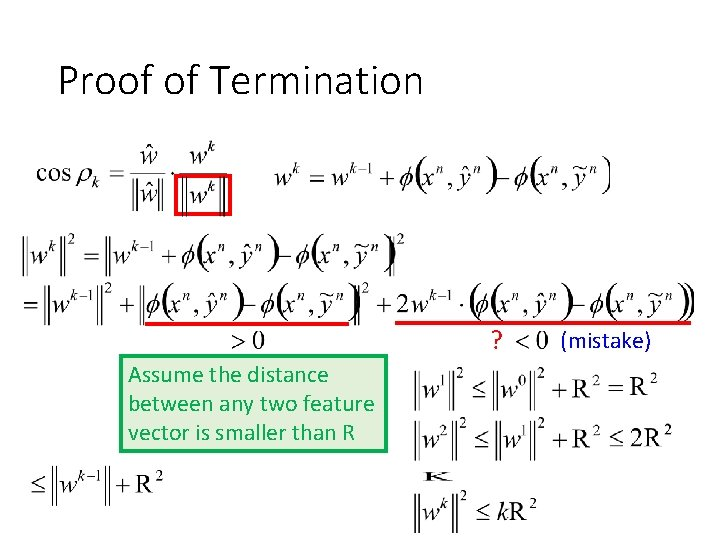Proof of Termination ? Assume the distance between any two feature vector is smaller than R (mistake)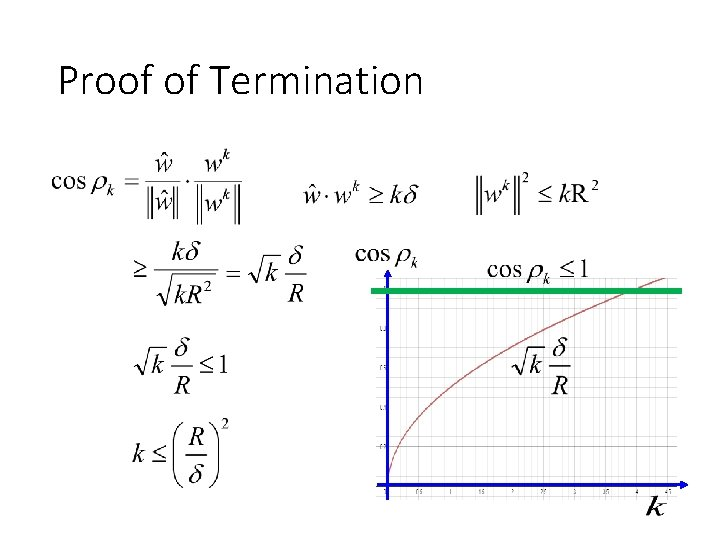Proof of Termination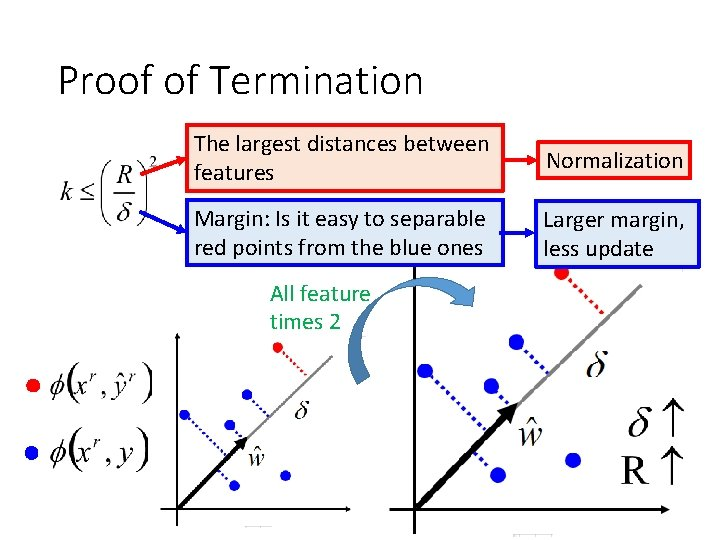Proof of Termination The largest distances between features Normalization Margin: Is it easy to separable red points from the blue ones Larger margin, less update All feature times 2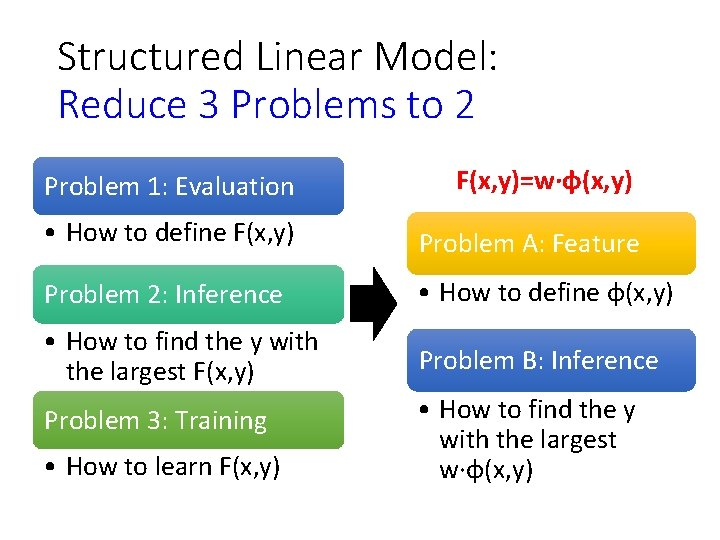Structured Linear Model: Reduce 3 Problems to 2 Problem 1: Evaluation F(x, y)=w·φ(x, y) • How to define F(x, y) Problem A: Feature Problem 2: Inference • How to define φ(x, y) • How to find the y with the largest F(x, y) Problem B: Inference Problem 3: Training • How to learn F(x, y) • How to find the y with the largest w·φ(x, y)Graphical Model A language which describes the evaluation functionStructured Learning We also know how to involve hidden information. Problem 1: Evaluation • What does F(x, y) look like? Problem 2: Inference • How to solve the “arg max” problem Problem 3: Training • Given training data, how to find F(x, y) Structured SVM, etc.Difficulties Difficulty 1. Evaluation Graphical Model Hard to figure out? Hard to interpret the meaning? Difficulty 2. Inference Gibbs Sampling We can use Viterbi algorithm to deal with sequence labeling. How about other cases?Graphical Model Graph • Define and describe your evaluation function F(x, y) by a graph • There are three kinds of graphical model. • Factor graph, Markov Random Field (MRF) and Bayesian Network (BN) • Only factor graph and MRF will be briefly mentioned today.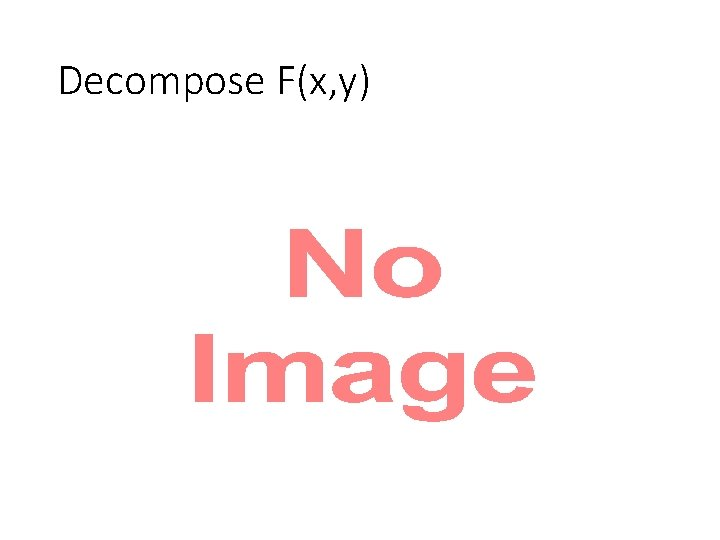Decompose F(x, y) •Decomposable x and y • x and y are decomposed into smaller components POS Tagging x 1 x: x 3 x 4 John saw the saw. y 1 y: x 2 {word} PN y 2 V y 3 y 4 D N x: x 1 x 2 x 3 x 4 y: y 1 y 2 y 3 y 4 {tags}Factor Graph x 1 X factor a Each factor influences some components. Each factor corresponds to a local function. y 1 x 2 factor b Y y 2 factor c Larger value means more compatible. You only have to define the factors. The local functions of the factors are learned from data.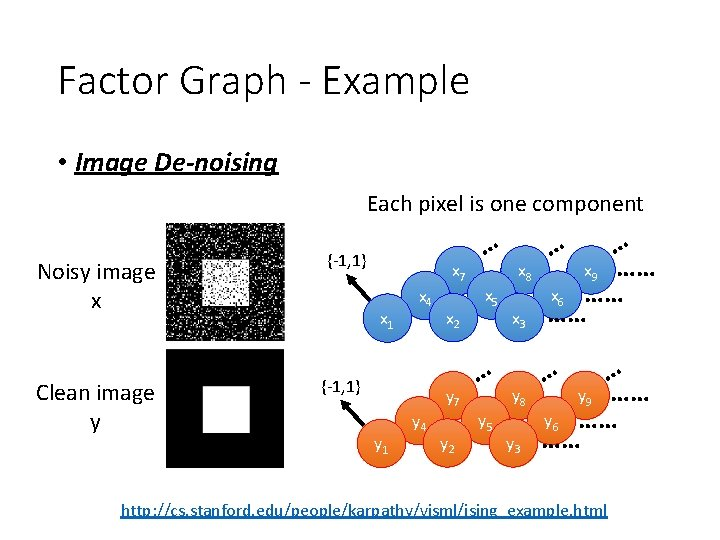Factor Graph - Example • Image De-noising Each pixel is one component Noisy image x Clean image y {-1, 1} x 7 x 1 x 4 {-1, 1} x 2 y 7 y 1 y 4 y 2 … x 5 … y 5 x 8 x 3 y 8 y 3 … x 9 …… x 6 …… …… … … y 9 …… y 6 …… …… … http: //cs. stanford. edu/people/karpathy/visml/ising_example. html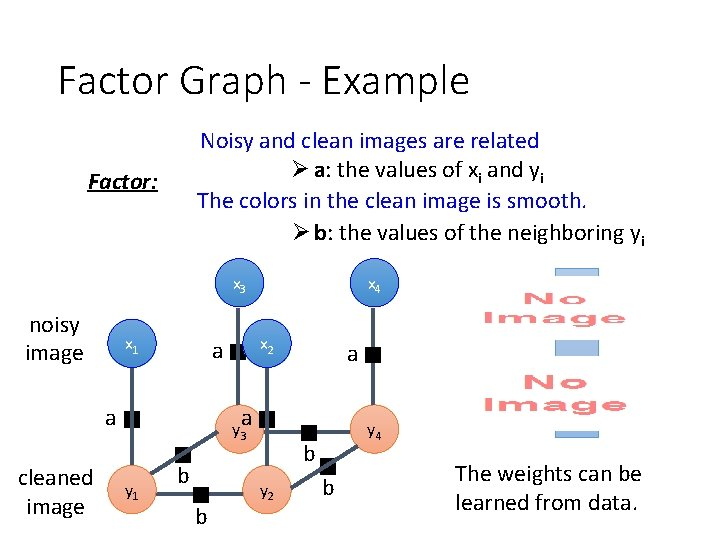Factor Graph - Example Noisy and clean images are related Ø a: the values of xi and yi The colors in the clean image is smooth. Ø b: the values of the neighboring yi Factor: x 3 noisy image x 1 cleaned image x 2 a a x 4 a a y 3 y 1 b b y 4 b y 2 b The weights can be learned from data.Factor Graph - Example Noisy and clean images are related Ø a: the values of xi and yi The colors in the clean image is smooth. Ø b: the values of the neighboring yi Factor: x 3 noisy image x 1 cleaned image x 2 a a x 4 a a y 3 y 1 b b y 4 b y 2 b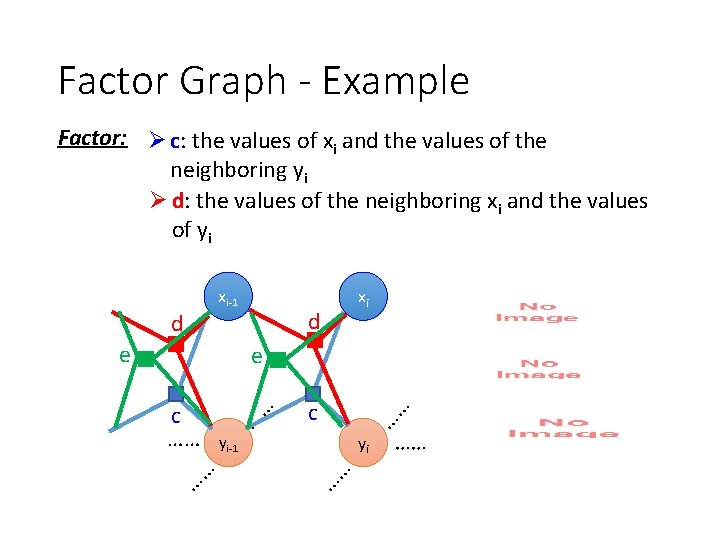Factor Graph - Example Factor: Ø c: the values of xi and the values of the neighboring yi Ø d: the values of the neighboring xi and the values of yi xi-1 d e d xi e …… yi-1 yi …… …… …… c ……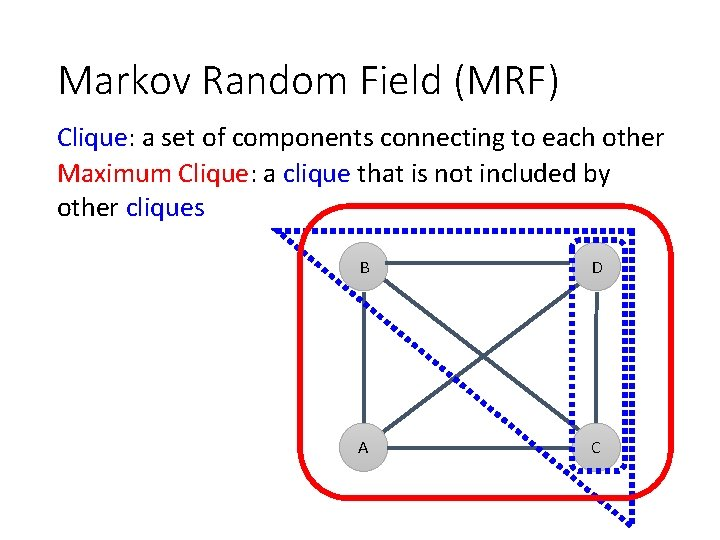Markov Random Field (MRF) Clique: a set of components connecting to each other Maximum Clique: a clique that is not included by other cliques B D A CEach maximum clique on the graph corresponds to a factor MRF Factor Graph MRF A A B B B A A C B D A C A B B C C DMRF Factor Graph b a d c Evaluation Function e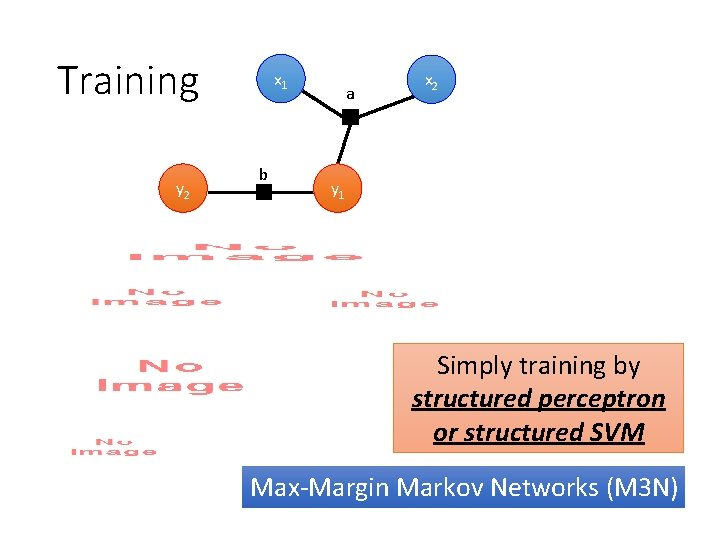Training y 2 x 1 b a x 2 y 1 Simply training by structured perceptron or structured SVM Max-Margin Markov Networks (M 3 N)Training x 1 b y 2 y 1 y 2 +1 +1 w 1 +1 -1 w 2 -1 +1 w 3 -1 -1 w 4 a y 1 x 2Now can you interpret this?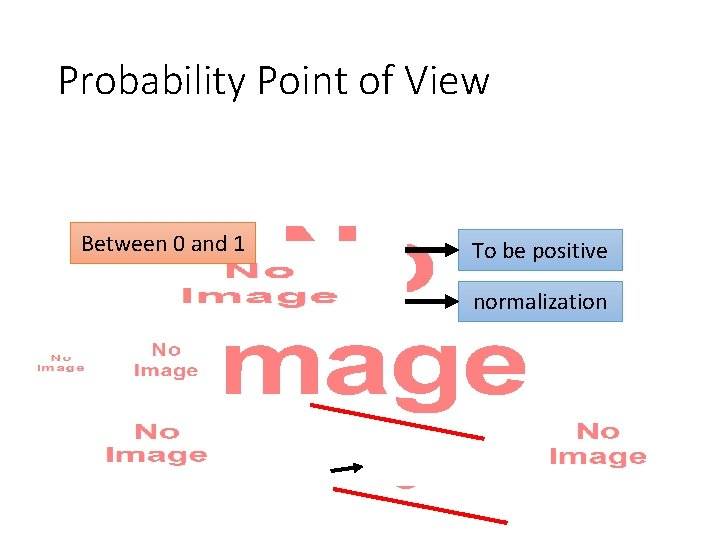Probability Point of View • Between 0 and 1 To be positive normalization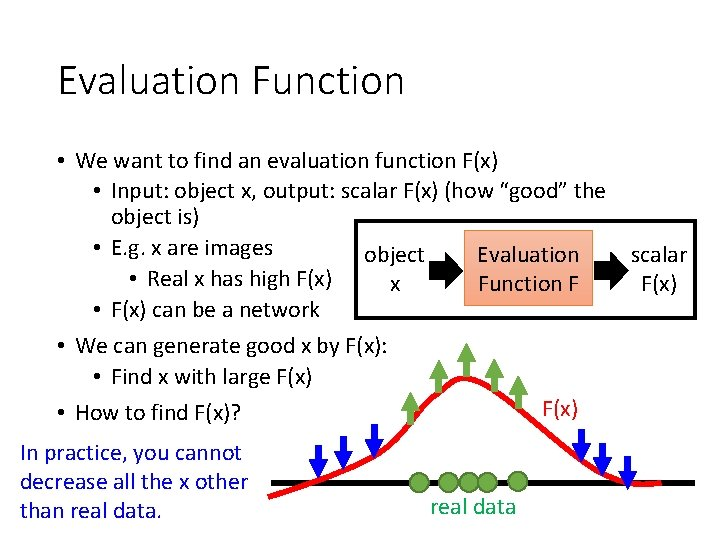Evaluation Function • We want to find an evaluation function F(x) • Input: object x, output: scalar F(x) (how “good” the object is) • E. g. x are images Evaluation object scalar • Real x has high F(x) Function F x F(x) • F(x) can be a network • We can generate good x by F(x): • Find x with large F(x) • How to find F(x)? In practice, you cannot decrease all the x other than real dataEvaluation Function - Structured Perceptron • Can be an issue We are done!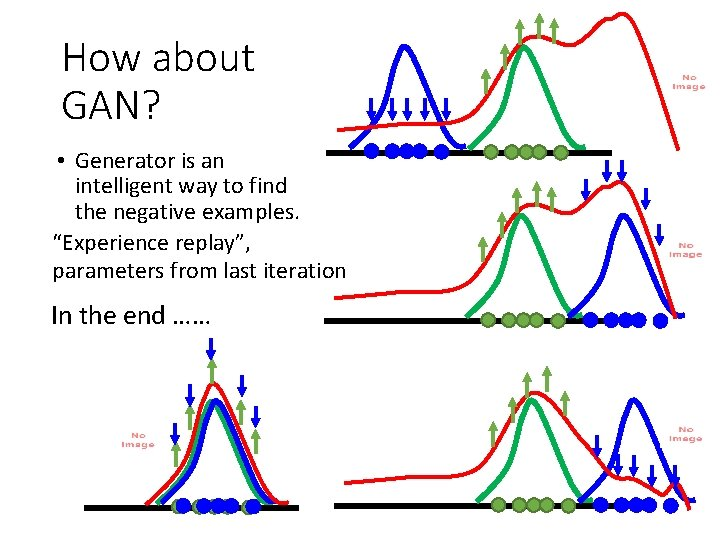How about GAN? • Generator is an intelligent way to find the negative examples. “Experience replay”, parameters from last iteration In the end ……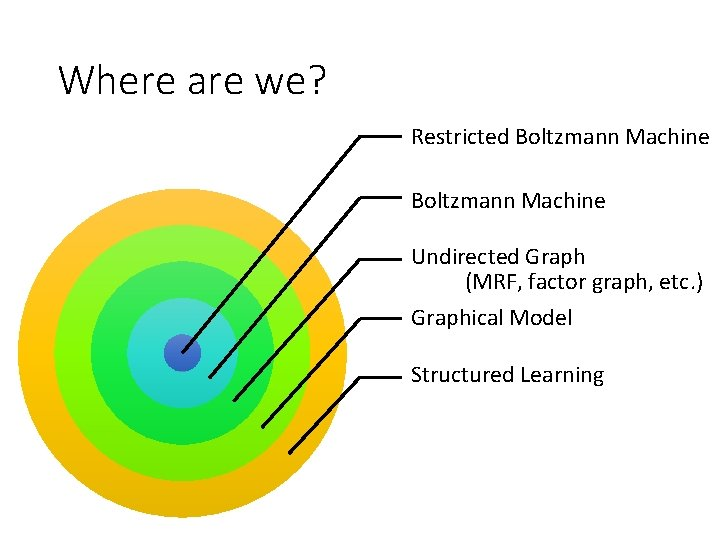Where are we? Restricted Boltzmann Machine Undirected Graph (MRF, factor graph, etc. ) Graphical Model Structured Learning